# Noise in photographic images

Current Documentation
View previous documentation
View legacy documentation
All documentation versions

 Dynamic Range – a general introduction with links to Imatest modules that calculate it. Color/Tone / eSFR ISO Noise – including chroma, sensor (RAW), and visual noise Temporal Noise – comparing the two-image and multi-image measurements

### Introduction

Noise is a random variation of image density, visible as grain in film and pixel level variations in digital images. It is a key image quality factor; nearly as important as sharpness. It is closely related to dynamic range— the range of brightness a camera can reproduce with reasonably good Signal-to-Noise Ratio (SNR). Since it arises from basic physics— the photon nature of light and the thermal energy of heat— it will always be present. The good news is noise can be extremely low in digital cameras with large pixels (pixel pitch ≥ 3 μm). But noise can get ugly in compact digital cameras with tiny pixels, especially at high ISO speeds, and noise reduction software can cause a visible loss of fine detail (texture).

In most cases noise is perceived as a degradation in quality. But some Black & White photographers like its graphic effect: Many favored 35mm Tri-X film. (Film grain has very different statistics from digital noise— it’s multiplicative rather than additive, and its spectrum is dependent on density.) Pointillist painters, most notably George Seurat, created images from “noise” (specks of color) by hand; a task that can be accomplished in seconds today with Photoshop plugins. But noise is undesirable for most pictorial and technical imaging.

With just one exception, noise is measured from flat patches in test charts (there are many to choose from) or from flat field images. The exception is noise measured in a sinusoidal Siemens Star pattern, used to calculate Camera Information Capacity (a camera figure of merit that combines noise and sharpness).

Noise is measured by several Imatest modules: Color/Tone Interactive, Color/Tone AutoeSFR ISO, Colorcheck, Stepchart, Image Statistics, and to a limited degree in SFR, SFRplus, and Uniformity. Color/Tone Interactive, Color/Tone Auto, and eSFR ISO have the most comprehensive noise measurements.

Noise is typically measured as RMS (Root Mean Square) noise, which is identical to the standard deviation of the flat patch signal S.

$$RMS\ Noise = \sigma(S)$$, where σ denotes the standard deviation. RMS is used because $$Noise\ Power = (RMS\ Noise)^2$$.

S can be the signal in any one of several color channels: R, G, B, Y (luminance, typically 0.2125 R + 0.7154 G + 0.0721 B),  raw channels (R, Gr, B, Gb), or a derived channel such as R-Y or B-Y (used for chroma noise). L*, a*, b*, or others. See Color/Tone/eSFR ISO noise measurements.

Signal-to-Noise Ratio (SNR or S/N)— Since noise is only meaningful in relationship to a signal, SNR is often more useful than noise itself. SNR can be defined in many ways, depending on how Signal S is defined. For example, S can be an individual patch pixel level or the pixel difference corresponding to a specified scene density range (1.45 or 1.5 are often used for this purpose) or a low contrast object (for Contrast Resolution SNR). It is important to know precisely how SNR is defined whenever it is discussed. SNR can be expressed as a simple ratio (S/N) or in decibels (dB), where SNR (dB) = 20 log10(S/N). Doubling S/N corresponds to increasing SNR (dB) by 6.02 dB. Most Imatest modules have several noise and SNR measurements, some simple and some detailed.

In digital cameras noise consists of two parts: fixed noise (sensor dark current noise and thermal (resistive) noise) and shot (photon) noise, which increases with the square root of the mean number of photons striking the pixels.

### Noise measurements

Imatest has a method for correcting noise measurements made from unevenly illuminated patches, which can result is serious measurement errors. Since uneven illumination is very common, we describe the correction process in the box below to strongly emphasize it.

### Correcting noise in patches with nonuniform illumination (caused by vignetting, etc.)

Noise measurements may deviate from the Noise = N = σ(S) formulation when the patch used to measure noise is unevenly illuminatedwhich happens frequently. Uneven illumination increases the measured value of N above the amount caused by random noise itself, potentially leading to serious errors.

To account for this we calculate second order fits to the mean signal value in both the x and y-directions, then subtract the second order fits from the signal prior to calculating noise. Hence Imatest noise measurements made with nonuniformity correction are often lower than plain standard deviations.

The Imatest calculation can be set in a dropdown menu in Options II and in settings windows for modules that calculate noise. We recommend New noise uniformity correction, but there is an option for turning the nonuniformity correction off (only recommended for comparison with external standard deviation calculations).

When significant nonuniformity is present, it can dominate noise measurements if nonuniformity correction is not applied. The noise measurement can become strongly dependent on the size of the region (ROI). When nonuniformity correction is applied, it becomes relatively independent of region size.

For this reason, noise nonuniformity correction is absolutely critical for accurate, reliable noise measurements.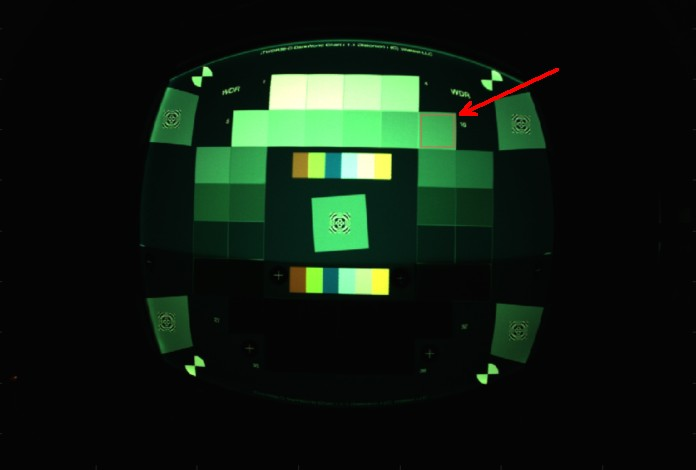We illustrate this with a vignetted image of an Imatest 36-patch Dynamic Range chart, shown greatly reduced on the right, using patch 10 (the last patch on the second row, indicated by the red arrow ). Patch 10 was chosen because the green channel in patches 1-8 were saturated.

The green channel in lower left of patch 10 (closest to the image center) has a mean value of 205 (of 255). The mean value on the upper right is 160: 20% lower. Here are Image Statistics results for two different crops of the region, without and with nonuniformity correction. Similar results are observed with Color/Tone without and with nonuniformity correction (NU corr).

 NU Corr ROI size SNR (db) Green Δ(On−Off) (dB) Off 20×19 32.2 Off 68×66 24.1 On 19×20 37.3 5.1 On 67×64 36.9 12.8

SNR with NU corr On is higher and also more consistent. SNR without NU corr is lower and strongly affected by region size: the smaller the region, the higher the SNR. The 13 dB difference for SNR with and without NU corr for the ~66×66 regions (which includes most of the patch) is extremely significant, as is the difference between the large and small ROIs with NU corr off.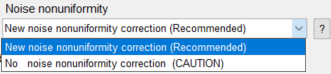Noise measurements should correlate, where possible, with perceived appearance and be simple enough to interpret with minimal difficulty. There are a number of cases with additional requirements.

• For Dynamic Range calculations, noise should be referenced to the original scene so the measurement is not affected by the tonal response (gamma-encoding), which is applied in the RAW conversion process.
• For sensor performance calculations, noise should be measured from raw images (undemosaiced where possible).

Color/Tone Interactive, Color/Tone Auto, Stepchart, and Colorcheck include the following noise displays:

Noise as a function of pixel level or exposure, expressed in

• Pixels, normalized to the pixel difference corresponding to a scene density range of 1.5 for Stepchart (comparable to the density range of 1.45 for the GretagMacbeth ColorChecker) or the White – Black zones in the ColorChecker (row 3, patches 1 – 6; density range = 1.45). Without this normalization, noise is a function of RAW converter response curve; it increases with converter contrast.
• Pixels, normalized to the maximum pixel value: 255 for 8/24-bit files.
• Pixels (maximum of 255 for 8/24-bit files)
• f-stops (or EV or zones; a factor of two in exposure), i.e., referenced to the original scene. Noise measured in f-stops corresponds closely to human vision. See f-stop noise, below.

Noise as a simple (average) number

• A good single number for describing noise is the average noise of the Y (Luminance) channel, measured in pixels (normalized scene density difference of 1.5), shown in the lower plot in the figures, below. The lightest and darkest zones, representing chart densities greater than 1.5 or less than 0.1 are excluded from the average.

S/N or SNR (dB) as a function of pixel level or exposure, where S is the pixel level of the individual test chart patch.

S/N or SNR (dB) as a simple (average) number.

• A good single number for defining SNR is the White – Black SNR, SNR_BW, where S is the difference in pixel levels between the White and Black patches in the GretagMacbeth ColorChecker in Colorcheck (density range of 1.45), or the pixel difference corresponding to a chart (scene) density difference of 1.5 in Stepchart. Noise is measured in a gray patch with density around 0.7. SNR_BW was developed to reduce the effect of image contrast (resulting from image processing, i.e., the application of a gamma curve) on the noise measurements.
$$SNR_{BW} = 20 \log_{10}\bigl( \frac{S_{WHITE} – S_{BLACK}}{N_{MID}} \bigr)$$

a noise spectrum plot, described below.

Uniformity and Uniformity Interactive display a spatial map of noise when Fine detail or Grid plot is selected.

Color/Tone Interactive, Color/Tone Auto, and eSFR ISO contain the most comprehensive noise analysis and displays. They measure Noise, S/N (as a ration), and SNR (dB) for a variety of channels, including RGB (original data), Chroma, CIELAB (L*a*b*), all pixels (for characterizing sensor noise from RAW images), F-stop (scene-referenced) noise (for Dynamic Range measurements), ISO 15739 Visual noise and Dynamic range, CPIQ visual noise, and YUV. A complete table and detailed descriptions can be found here.

Image Statistics can measure detailed noise statistics — including histograms and noise spectra — from any region of any image.

### Noise appearance

The appearance of noise is illustrated in the stepchart images on the right. Noise is usually measured as an RMS (root mean square) voltage. The mathematics of noise is presented in a green box at the bottom of this page. The patches in columns (A)-(C) are simulated. They are assumed to have a minimum density of 0.05 and density steps of 0.1, identical to the Kodak Q-13 and Q-14. They have been encoded with gamma = 1/2.2 for optimum viewing at gamma = 2.2 (the Windows/Internet standard). Strong noise— more than you’d find in most digital cameras— has been added to columns (A) and (B). Column (C) is noiseless. The fourth column (D) contains an actual Q-13 stepchart image taken with the Canon EOS-10D at ISO 1600: a very ISO high speed. Noise is visible, but low for such a high ISO speed (thanks, no doubt, to software noise reduction).

The noise in (A) is constant inside the sensor, i.e., before gamma encoding. When it is encoded with gamma = 1/2.2, contrast, and hence noise, is boosted in dark areas and reduced in light areas. The On Semiconductor publication, CCD Image Sensor Noise Sources, indicates that this is not a realistic case. Shot (photon) noise causes sensor noise to increase with brightness. The noise in (B) is uniform in the image file, i.e., its value measured in pixels is constant. This noise must therefore increase with brightness inside the sensor (prior to gamma encoding), and hence is closer to real sensor behaviour than (A). Noise appears relatively constant except for the darkest zones, where it’s not clearly visible. Noise is often lower in the lightest zones, where a tonal response “S” curve superimposed on the gamma curve (or saturation) reduces contrast, hence noise. For this reason the middle zones— where noise is most visible— are used to calculate the average noise: a single number used to characterize overall noise performance. We omit zones where the density of the original chart (hence display density in an unmanipulated image) is greater than 1.5 or less than 0.1.

Characteristic Stepchart results are shown below.

 (A) Const. sensor noise (B) Const. pixel noise (C) No noise (D) Canon EOS-10D ISO 1600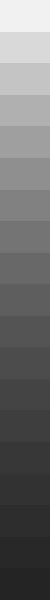#### Column (D): Canon EOS-10D at ISO 1600

The upper plot shows the tonal response with a slight “S” curve superimposed on the gamma curve (which would be a straight line in this log-log plot).

The middle plot shows f-stop (scene-referenced) noise (the inverse of scene-referenced SNR). Dynamic range is shown for several quality levels (scene-referenced SNR = 20, 12, 6, and 0 dB).

The lower plot shows the normalized pixel noise. It increases in the dark regions due to a combination of gamma-encoding and the high ISO speed. Digital cameras achieve high ISO speed by amplifying the sensor output, which boosts noise, particularly in dark regions. This curve looks different for the minimum ISO speed: noise values are much lower and subject to more statistical variation.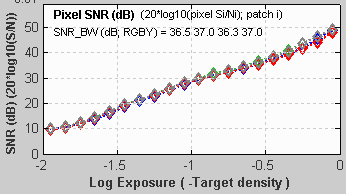This is SNR in dB for the Canon EOS-10D at ISO 1600. SNR improves by about 6 dB for each doubling of exposure (0.301 density units); roughly 20 dB per decade (1 density unit), which is what would be expected for constant sensor noise. (At ISO 1600, signal and noise are multiplied by a factor of 16 over the baseline ISO 100 values, meaning the maximum signal is only 1/16 as large. In this range of illumination, shot noise is not prominent. This curve would be dramatically different at lower ISO speeds, where shot noise has an effect.)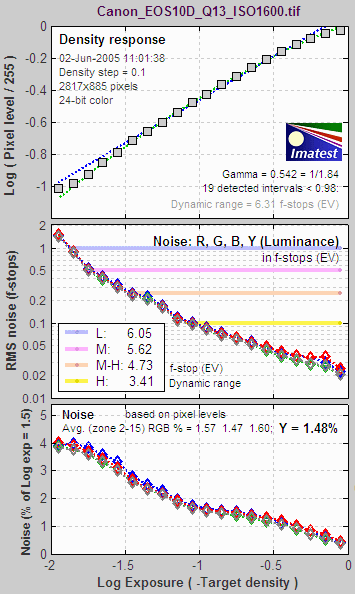### Noise summary

#### There are two basic types of noise.

Temporal noise can be reduced by signal averaging, which involves summing N images, then dividing by N. This is an option for all Imatest analysis modules when several image files are selected (you can also analyze the individual files separately). Summing N individual images increases the summed signal pixel level (voltage) by N. But since temporal noise is uncorrelated, noise power (rather than voltage or pixel level) is summed. Since voltage is proportional to $$\sqrt{\text{power}}$$, the noise pixel level (which is proportional to noise voltage) increases by $$\sqrt{N}$$. Signal-to-noise ratio (S/N or SNR) improves by $$N / \sqrt{N} = \sqrt{N}$$. S/N is improved by a factor of 2 (6.01 dB) for 4 images, 4 for 16 images, etc.

#### Several factors affect noise.

• Pixel size. Simply put, the larger the pixel, the more photons reach it, and hence the better the signal-to-noise ratio (SNR) for a given exposure. The number of electrons generated by the photons and the full-well (electron) capacity is proportional to the sensor area (as well as the quantum efficiency). Noise power is also proportional to the sensor area, but noise voltage is proportional to the square root of power and hence √area.
• Sensor technology and manufacturing. For a given pixel size, backside-illuminated (BSI) sensors have superior noise and SNR peroperties. We observed an improvement when we compared cameras in Shannon Information Capacity. An older technology issue was CMOS vs. CCD.  Until 2000 CMOS was regarded as having worse noise, but it has improved to the point where it almost completely dominates the industry. Other aspects of sensor design and manufacturing are gradually improving with time.
• ISO speed (Exposure Index) setting. Digital cameras control ISO speed by amplifying the signal (along with the noise) at the pixel output. The higher the ISO speed, the shorter the exposure (fewer electrons reach the sensor), and hence the worse the SNR. To fully characterize a sensor it should be tested at the lowest available ISO speed. For practical applications, performance at high ISO speeds is also of interest.
• Exposure time. Long exposures with dim light tend to be noisier than short exposures with bright light, i.e., reciprocity doesn’t work perfectly for noise. To fully characterize a sensor it should be tested at long exposure times (several seconds, at least).
• Digital processing. Sensors typically have ~12-bit analog-to-digital (A-to-D) converters. When an image is converted to an 8-bit (24-bit color) JPEG, noise increases slightly. The noise increase can be worse (“banding” can appear) if extensive image manipulation (dodging and burning) is required. Hence it is often best to convert to 16-bit (48-bit color) files. Output file bit depth makes little difference in the measured noise of unmanipulated files.
• Raw conversion. In-camera raw converters, used to create camera JPEG files, usually apply noise reduction (lowpass filtering) in smooth areas and sharpening near edges whether you want it or not; even if NR and sharpening are turned off. This affects noise measurements, making it difficult to measure the sensor’s true performance. Raw converters built into Imatest (dcraw for commercial files, Read Raw for binary files, or the Matlab demosaic function for special cases (Rawview)) minimally process images. Output from these converters is OK for noise measurements.

• Imatest subtracts gradual pixel level variations from the image before calculating noise (the standard deviation of pixel levels in the region under test). This removes errors that could be caused by uneven lighting. Nevertheless, you should take care to illuminate the target as evenly as possible.
• The target used for noise measurements should be smooth and uniform– grain (in film targets) or surface roughness (in reflective targets) should not be mistaken for sensor noise. Appropriate lighting (using more than one lamp) can minimize the effects of surface roughness.

### Temporal noise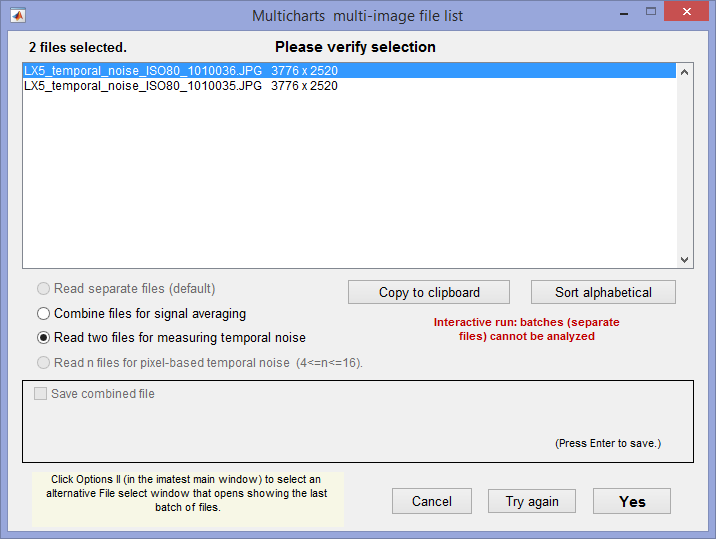Four Imatest modules—  Color/Tone Interactive, Color/Tone AutoColorcheck, and Stepchart— can measure temporal noise— noise that varies independently from image to image, with fixed-pattern noise omitted. It can be calculated by two methods:

1. the difference between two identical test chart images (the Imatest recommended method), and
2. the ISO 15739-based method, which where it is calculated from the pixel difference between the average of N identical images (≥ 8) and each individual image (Color/Tone Interactive and Color/Tone Auto-only).

(1) Difference method. In any of the modules, read two images. The window shown on the right appears. Select the Read two files for measuring temporal noise radio button.

The two files will be read and their difference (which cancels fixed pattern noise) is taken. Since these images are independent, noise powers add. For indendent images I1 and I2, temporal noise is

$$\displaystyle \sigma_{temporal} = \frac{\sigma(I_1 – I_2)}{\sqrt{2}}$$

In Color/Tone Interactive and Color/Tone Auto, temporal noise is displayed as dotted lines in Noise analysis plots 1-3 (simple noise, S/N, and SNR (dB)).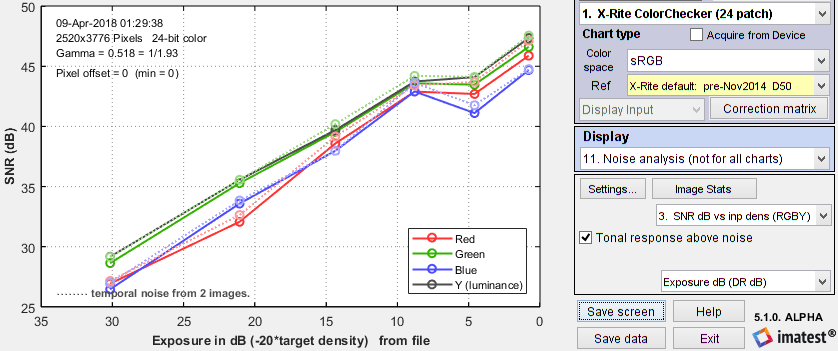SNR (dB) for Colorchecker chart: temporal noise shown as thin dotted lines.

(2) Multiple file method. From ISO 15739, sections 6.2.4, and 6.2.5. Currently we are using simple noise (not yet scene-referred noise). Select between 4 and 16 files. In the multi-image file list window (shown above) select Read n files for temporal noise. Temporal noise is calculated for each pixel j using

$$\displaystyle \sigma_{diff}(j) = \sqrt{\frac{1}{N} \sum_{i=1}^N (X_{j,i} – X_{AVG,j})^2} = \sqrt{\frac{1}{N} \sum_{i=1}^N X_{j,i}^2 – \left(\frac{1}{N} \sum_{i=1}^N X_{j,i}\right)^2 }$$

The latter expression is used in the actual calculation since only two arrays, $$\sum X_{j,i} \text{ and } \sum X_{j,i}^2$$, need to be saved. Since N is a relatively small number (between 4 and 16, with 8 recommended), it must be corrected using formulas from Identities and mathematical properties in the Wikipedia standard deviation page.  $$s(X) = \sqrt{\frac{N}{N-1}} \sqrt{E[(X – E(X))^2]}$$.

$$\sigma_{temporal} = \sigma_{diff} \sqrt{\frac{N}{N-1}}$$

We currently recommend the difference method (1) because our experience so far has shown no advantage to method (2), which requires many more images (≥ 8 recommended), but allows fixed pattern noise to be calculated at the same time. There is a detailed comparison of the methods in Measuring Temporal Noise.

### Measuring noise in raw versus demosaiced images

A customer recently questioned whether is was appropriate to measure noise in demosaiced images, where the signal in each color channel (R, G, B) is influenced by data in each of the raw color channels (R, Gr, B, and Gb). The quick answer is yes. We recommend measuring noise and SNR in the image (raw or demosaiced) that most closely resembles the use case. Raw images are best when measuring sensor performance, but demosaiced images are fine in most cases. This excellent question led us into a deep dive, which you can see by clicking the button below.

In demosaiced images, the signal in each color channel (R, G, B) is influenced by data in each of the raw color channels (R, Gr, B, and Gb), each of which has a quarter as many pixels as the demosaiced R, G, and B channels. Because the differences between the raw and demosaiced channels, we cannot expect identical noise measurements.

To answer the question we used an image of the Imatest 36-patch Dynamic Range chart, acquired on a 16-Megapixel Micro Four-Thirds camera at ISO 160 (3.77 μm pixel pitch). Both RAW and JPEG images were captured. The RAW file was converted to DNG (raw) format, then read into Rawview (using the Options II setting, “3. Read DNG files directly then linearize & correct…”), which enabled us to save a full-color RGB version of the image (using the Matlab demosaic routine), as well as individual raw channels (R, Gb, B, and Gb). This was done to ensure that all files were processed identically (and minimally– no noise reduction, sharpening, gamma encoding or color correction).

We have long been aware that demosaicing affects the frequency spectrum of the patches. Results, displayed by Image Statistics, are from patch 4 (which had statistics typical of most patches) of the demosaiced image and from two of the four Bayer raw channels (the camera had a GRBG = Red in R2C1 pixel arrangement).

The large response at low frequencies is apparently caused by small amounts of illumination nonuniformity. The frequency spectrum in the important region between 0.1 and 0.5 Cycles/Pixel (the Nyquist frequency, fNyq), characterized by the decrease in spectral response, is significant. For the two raw images (R1C1 (Gr) and R2C1 (Blue)), the response (apart from the noise) is nearly flat. But for the demosaiced image, it drops by about 0.35 density units, apparently caused by demosaicing (we’ve seen this before).

Note that fNyq in cycles/physical distance (such as mm) is different for raw and demosaiced images: because raw pixel spacing is 2× demosaiced pixel spacing, fNyq (cycles/mm) is only half as large for the raw image. For this reason the decrease in spectral response from 0.05 to 0.25 C/P in the demosaiced image (shown in parentheses in the table below) may be more appropriate for comparing with the raw spectral response.

 Channel SNR (dB) Y (demosaiced) 37.4 R (demosaiced) 32.9 G (demosaiced) 37.9 B (demosaiced) 31.7 Gr  (R1C1) 36.5 Blue (R2C1) 33.8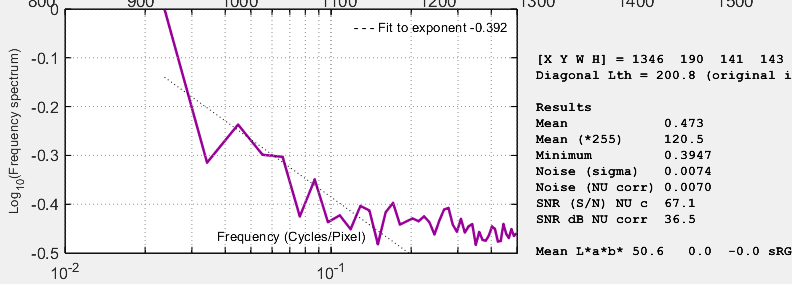Frequency spectrum of R1C1 (Gr) image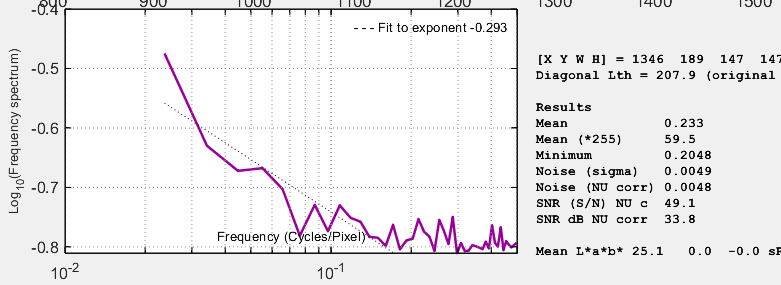Frequency spectrum of R2C1 (Blue) image

It is difficult to draw a clear conclusion from SNR results because measured SNR for raw and demosaiced images are close. For the demosaiced image, SNR is slightly higher for the Green channel and slightly lower for the Red and Blue channels. Differences are small — around 1 dB. For this reason we recommend measuring noise and SNR in the image (raw or demosaiced) most closely connected with the use case. Raw images are best when measuring sensor performance, but demosaiced images are fine in most cases.

The noise spectrum is, however, quite different in raw and demosaiced images. It drops off more quickly in demosaiced images— apparently the direct result of the demosaicing process. The noise spectrum of the raw images is of considerable interest because it would be flat (as we’ve seen) if the sensor pixels were completely independent. This may not be the case for very small pixels (more work needed).

We did not include results from the in-camera JPEG file of the same image because the JPEG appeared to have strong noise reduction (lowpass filtering) and color correction (which also affects noise). For the record, SNR for the R, G, B, and Y channels was 44.8, 48.0, 41.0, and 49.5 dB, and the response decrease from 0.1 to 0.5 C/P was 0.85: much larger than the minimally-processed undemosaiced image show above, and a very clear indicator of software noise reduction (which causes a loss of texture or fine detail).

### Noise frequency spectrum

In addition to an amplitude distribution, noise is characterized by a frequency spectrum, calculated by taking the Fourier transform of the spatial image. The spectrum is closely related to appearance. Two spectra are shown below. The first is for the image from column (B), above, which contains simulated white noise. The second is for the image in column (D), above, taken with the Canon EOS-10D at ISO 1600. The images shown below have been magnified 2X (using the nearest neighbor resizing algorithm) to emphasize the pixel distribution. They are approximations to the images used to calculate the spectra (though not the exact images).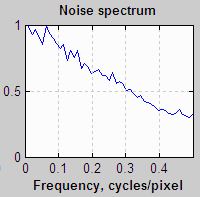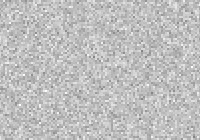White noise, enlarged 2X (nearest neighbor)Blurred noise, enlarged 2X (nearest neighbor)

White noise (unfiltered electronic noise is white) has two key properties. 1. The values of neighboring pixels are uncorrelated, i.e., independent of one-another. 2. Its spectrum is flat. The white noise spectrum (above, left) shows statistical variation and a small peak at 0.25 cycles/pixel, probably caused when the image in column (B) was resized for display. For spectral (non-white) noise, neighboring pixels are correlated and the spectrum is not flat. The spectrum and image (above, right) are the result of blurring (also called smoothing or lowpass filtering), which can result from two causes

1. The Bayer sensor demosaicing algorithm in the RAW converter causes the noise spectrum to drop by about half at the Nyquist frequency (0.5 cycles/pixel), and
2.  Noise reduction (NR) software lowpass filters noise, i.e., reduces high frequency components. With the widely-used bilateral filter (which can be simulated with the Image Processing module), NR uses a threshold that prevents portions of the image near contrast boundaries from blurring.

But NR comes at a price: detail with low contrast and high spatial frequencies (texture) can be lost. This causes the “plasticy” appearance sometimes visible on skin. Some people love it (plastic surgeons make a lot of income); I don’t This loss can be quantified with Imatest Log F-Contrast measurements.The visibility of noise depends on the noise spectrum, though the exact relationship is complex. Noise at high spatial frequencies may be invisible in small prints (low magnifications) but very damaging in large prints (large magnifications). Because of the complex nature of the relationship, Kodak established a subjective measurement of grain (i.e., noise) called Print Grain Index (Kodak Technical Publication E-58)Visual noise measurements are intended to predict the visual effect of noise, based on noise spectrum and viewing conditions.

Sharpening and unsharp masking (USM) are the inverse of blurring. They boost portions of the spectrum and cause neighboring pixels to become negatively correlated, i.e., they exaggerate the differences between pixels, making the image appear noisier. USM can be applied with a threshold that restricts sharpening to the vicinity of contrast boundaries. This prevents noise from degrading the appearance of smooth areas like skies. In images taken with poor quality lenses (or misfocused or shaken), the image is lowpass filtered (blurred) but the noise is not. Some sharpness loss can be recovered with sharpening or USM, but noise is boosted in the process. That’s why good lenses are important, even with digital sharpening.

### ISO 15739 noise and SNR

The updated ISO 15739:2013 standard has several definitions for noise, SNR, and Dynamic Range that are similar to f-stop noise, but with some important differences. The ISO definition of Signal-to-Noise Ratio (§6.2.3-6.2.5 and Annex D) is

$$SNR = Q = gL / \sigma$$

where  σ = noise in pixels, L = luminance (linear), and g is the first derivative of the OECF (pixel level vs. luminance curve).

$$g = d(\text{pixel}) / dL$$        Though it isn’t obvious from the standard, L must be scaled (multiplied) by Lref, as defined in §6.2.2. The numeric version this equation is given in Annex D.

When ISO 15739 SNR is displayed in Color/Tone Interactive, Color/Tone Auto, or eSFR ISO, it can be compared with the older Imatest f-stop-based (scene-referenced) noise calculation. Results are similar (though not identical).

 (Obsolete section: kept for reference) Definitions:      Pxl = pixel level (same as OL = output level in the ISO standard.)      σpx = noise in pixels (note: standard deviation σ is equivalent to RMS noise.)      L = Illumination or exposure level. (Units will not be important.)      f-stops = log2L      f-stop noise = σfst = σpx /(d(Pxl)/d(f-stops)) = σpx /(d(Pxl)/d(log2L))      f-stop SNR = SNRfst = 1/σfst = (d(Pxl)/d(log2L)) / σpx We will apply the equation, d(logb(x))/dx = 1/(x ln(b)), where ln(2) = 0.6931 = 1/1.4427. Now, from the ISO standard, Incremental gain = gI = d(Pxl)/dL (Note linear units) = d(Pxl)/d(log2L) × d(log2L) / dL = 1.442(d(Pxl)/d(log2L)) / L Appendix D of the ISO 15739 standard defines total Signal-to-Noise Ratio as SNRISO = L gI / σpx = 1.4427 L (d(Pxl)/d(log2L)) / (L σpx) = 1.4427 (d(Pxl)/d(log2L))/σpx which leads to      SNRISO = 1.4427 SNRfst SNRISO is larger than SNRfst by a factor of 1.4427, or equivalently, 3.18 dB.

### Appendix I: F-stop noise, scene-referenced SNR, and Dynamic Range

This material is in an Appendix because scene-referenced noise is used for Dynamic Range calculations, and hence is slightly outside the main topic of this page. It is repeated in

#### F-stop noise and scene-referenced SNRThe human eye responds to relative luminance differences. That’s why we think of exposure in terms of zonesf-stops, or EV (exposure value), where a change of one unit corresponds to a factor of 2 change in exposure.The eye’s relative sensitivity is expressed by the Weber-Fechner law,

Δ≈ 0.01 L  –or–  ΔL/L ≈ 0.01

where ΔL is the smallest luminance difference the eye can distinguish. This equation is approximate; effective ΔL tends to be larger in dark areas of scenes and prints due to visual interference (flare light) from bright areas.

Expressing noise in relative luminance units, such as f-stops, corresponds more closely to the eye’s response than standard pixel or voltage units. Noise in f-stops = Nf-stop is obtained by dividing the noise in pixels by the number of pixels per f-stop. (We use “f-stop” rather than “zone” or “EV” out of habit; any of them are OK.) Note that 1 f-stop = 0.301 Optical density units = 6.02dB (decibels) = log2(luminance).

Also note that Nf-stop is scene-referenced noise in (logarithmic) units of f-stops, and must be distinguished from linear scene-referenced noise, Nscene-ref, which has the same linear units as luminance (the scene signal).

 $$\text{F-stop noise } = N_{f-stop} = \displaystyle \frac{N_{pixels}}{d(\text{pixel})/d(\text{f-stop})} = \frac{N_{pixels}}{d(\text{pixel})/d(log_2 \text{luminance})}$$           $$= \displaystyle \frac{N_{pixels} \times ln(2) \times \text{luminance}}{d(\text{pixel})/d(\text{luminance})} ≅ \frac{N_{pixels} \times \text{luminance}}{d(\text{pixel})/d(\text{luminance})}$$ where Npixels is the measured noise in pixels and $$d(\text{pixel})/d(\text{f-stop})$$ is the derivative of the signal (pixel level) with respect to scene luminance (exposure) measured in f-stops = log2(luminance). We used $$d(log_a(x)) = dx / (x \, ln(a))$$. ln(2) = 0.6931 has been dropped to maintain backwards compatibility with older Imatest calculations. Noting that luminance (exposure) is the signal level of the scene,      $$\displaystyle \text{Scene-referenced noise} = N_{scene-ref} = \frac{N_{pixels}}{d(\text{pixel})/d(\text{luminance})} ≅ \frac{N_{f-stop}}{\text{luminance}}$$       $$\displaystyle \text{Scene-referenced Signal-to-Noise Ratio} = SNR_{scene-ref} = \frac{\text{luminance}}{N_{scene-ref}} = \frac{1}{N_{f-stop}}$$ Displays in Stepchart, Color/Tone Interactive, and Color/Tone Auto offer a choice between f-stop noise or Scene-referenced SNR (expressed as a ratio or in dB). Note that SNRscene-ref decreases as the slope of the tonal response curve decreases (often the result of flare light in dark patches).

The above-right image illustrates how the pixel spacing between f-stops (and hence d(pixel)/d(f-stop)) decreases with decreasing brightness. This causes f-stop noise to increase with decreasing brightness, visible in the figures above.

Since f-stop noise and scene-referenced SNR are functions of scene luminance, largely independent of image signal processing and fogging from flare light, they are an excellent indicators of real-world camera performance. They are the basis of Imatest Dynamic Range measurements.

Imatest has several types of Dynamic Range calculation, which are cross-referenced here.

 Dynamic range from a single transmissive chart image. Stepchart, Color/Tone Interactive, Mutitest A transmissive chart is such as the Imatest 36-patch Dynamic Range or HDR chart is required because reflective charts do not have sufficient tonal range. Dynamic range from multiple (differently exposed) images Dynamic Range module Uses CSV output of Stepchart of Color/Tone Auto for several differently exposed images. Usually used with reflective charts, but transmissive charts may also be used. ISO 15739 Dynamic Range from patch with density ≈ 2 Color/Tone Interactive, Color/Tone Auto, eSFR ISO Extrapolates Dynamic Range from a single patch with density ≈ 2. Raw sensor Dynamic Range Color/Tone Interactive, Color/Tone Auto Fits raw data to an equation from the EMVA 1288 standard, then extrapolates to find DR. The test chart does not have to have as large a tonal range as the DR, but transmissive charts with tonal range ≥ 3 are recommended.

Since scene-referenced noise and SNR are independent of the tonal response curve, they are used as the basis for Imatest Dynamic Range calculations, which are described elsewhere. Dynamic Range is the range of exposure (scene brightness) where quality exceeds a specified level, which we define as 20 dB for “high quality” down to 0 dB for “low quality”. Here is an illustration. The upper curve is the tonal response plot showing gamma. The lower plot is the scene-referenced noise, showing Dynamic Range at several quality levels.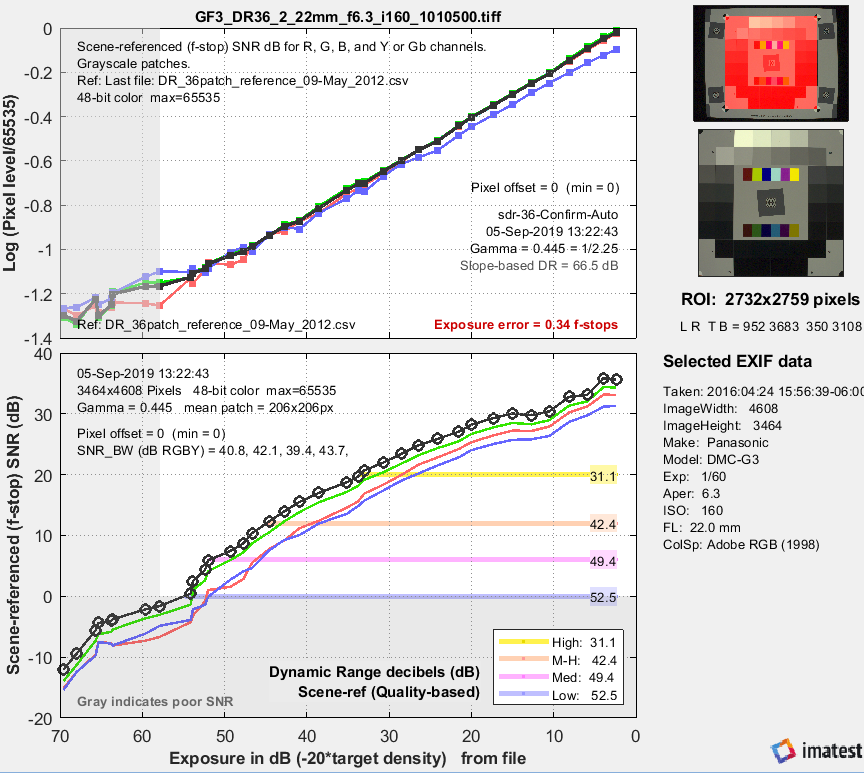Tonal response and scene-referenced SNR for an image converted from raw with dcraw.

### Appendix II: The mathematics of noise (just a taste)

 Amplitude distribution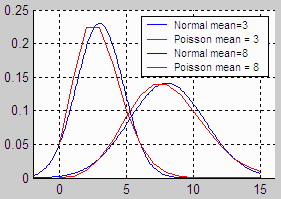In most cases, the pixel or density variations that comprise noise can be modeled by the normal distribution. This is the familiar Gaussian or “bell” curve (blue on the right) whose probability density function is $$f(x) = e^{-(x-a)^2 / 2 \sigma ^2} / \sqrt{2 \pi \sigma^2}$$ where a is the mean (average value) of x, and σ is the standard deviation. σ is proportional to the width of the distribution: for the normal distribution, about 68% of samples are between a ± σ; 95.5% between a ± 2σ; 99.7% between a ± 3σ, etc. For a set of N samples xi with mean xm , the standard deviation is $$\sigma = \sqrt{\frac{1}{N} \sum_{i = 1}^{N} (x_i – x_m)^2}$$  Noise is usually measured as RMS (root mean square) voltage or pixel level, where RMS is equivalent to standard deviation. RMS noise voltage is the square root of noise power. The value inside the square root is also called the variance. Noise varies with the pixel level, i.e., it differs in areas with different tonal values. The normal curve arises from a remarkable mathematical result called the central limit theorem: When a variable (such as voltage or pixel level) is affected by a large number of perturbations, the overall density function approaches the normal curve, regardless of the distributions of the individual perturbations. This is why the normal curve is by far the most common probability distrubution. But the normal distribution doesn’t apply in all situations. For low light levels (low photon counts), where the normal distribution could result in negative counts, the Poisson distribution (red in the illustration above) gives the correct result. $$f(s) = e^{-m} \frac{m^s}{s!}$$ where m is the mean, s ≥ 0 is an integer, and s! = “s factorial” = (s)(s-1)(s-2)…(1). The standard deviation is $$\sigma = \sqrt{m}$$. Shot (photon) noise, described in the Kodak publication, CCD Image Sensor Noise Sources, has Poisson statistics. For large values of m, the Poisson distribution approaches a normal distribution.